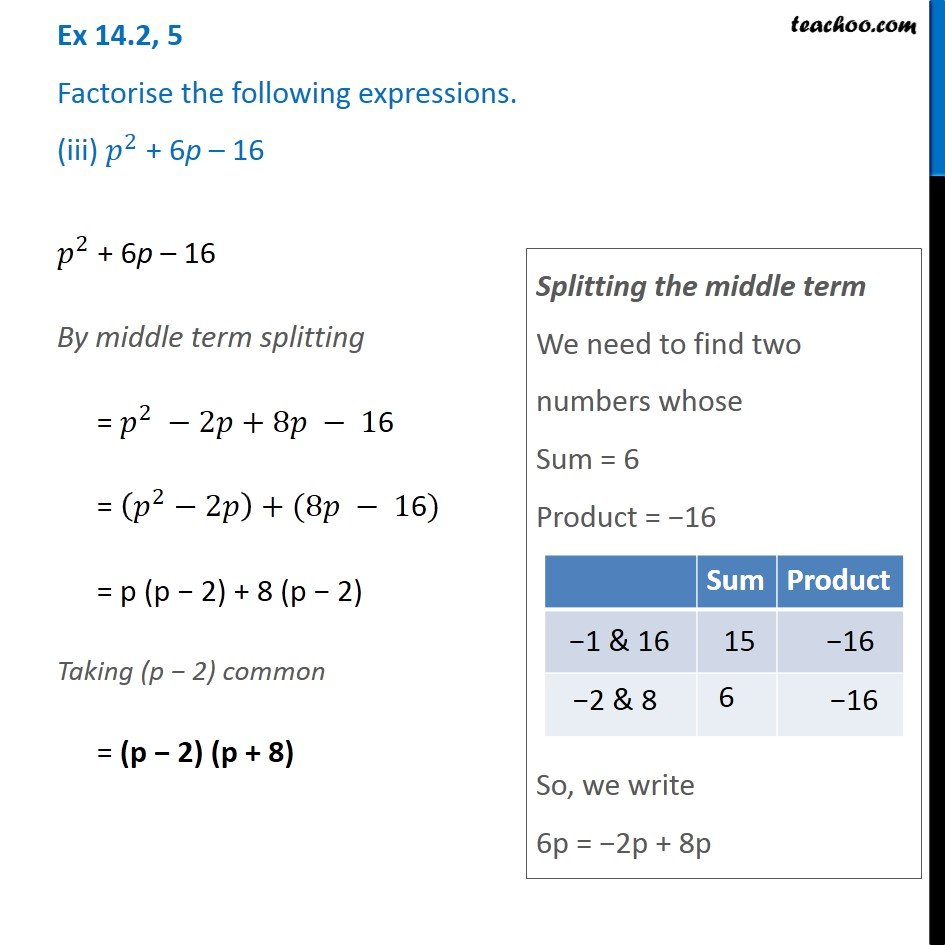Factorisation using splitting middle term

Chapter 14 Class 8 Factorisation
Concept wiseIntroducing your new favourite teacher - Teachoo Black, at only ₹83 per month

### Transcript

Ex 14.2, 5 Factorise the following expressions. (iii) 𝑝^2 + 6p – 16 𝑝^2 + 6p – 16 By middle term splitting = 𝑝^2 −2𝑝+8𝑝 − 16 = (𝑝^2−2𝑝)+(8𝑝 − 1"6") = p (p − 2) + 8 (p − 2) Taking (p − 2) common = (p − 2) (p + 8) Splitting the middle term We need to find two numbers whose Sum = 6 Product = −16 So, we write 6p = −2p + 8p